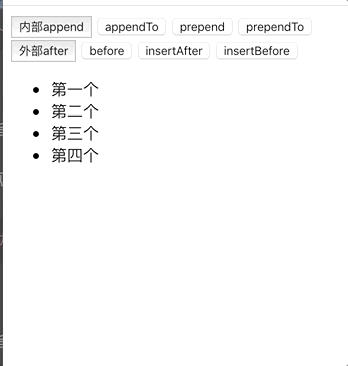# 「jQuery」节点的添加操作&节点的复制(浅拷贝&深拷贝)&节点删除&节点替换

## 节点添加

### 内部插入

#### append(content|fn)

``\$(元素).append("<b>Hello</b>");``

#### appendTo(content)

``要被添加到元素.appendTo("添加到这个元素里面");``

#### prepend(content)

``\$(元素).prepend("<b>Hello</b>");``

#### prependTo(content)

``要被添加到元素.prependTo("添加到这个元素里面");``

### 外部插入

#### after(content|fn)

``\$(元素).after("<b>Hello</b>");``

#### before(content|fn)

``\$("元素").before("<b>Hello</b>");``

#### insertAfter(content)

``被插入的元素.insertAfter("添加到这个元素里面");``

#### insertBefore(content)

``被插入的元素.insertBefore("添加到这个元素里面");````````<!DOCTYPE html>
<html lang="en">
<meta charset="UTF-8">
<meta name="viewport" content="width=device-width, initial-scale=1.0">
<title>添加节点操作-乔越博客</title>
<!-- 引入jquery库 -->
<script src="https://www.jq22.com/jquery/jquery-3.3.1.js"></script>
<script>
\$(function(){
\$("button").eq(0).click(function(){
var \$li = \$("<li>新增的</li>");
//内部添加 添加到最下面
\$("ul").append(\$li);
})
\$("button").eq(1).click(function(){
var \$li = \$("<li>新增的</li>");
//内部添加 添加到最下面
\$li.appendTo("ul")
})
\$("button").eq(2).click(function(){
var \$li = \$("<li>新增的</li>");
// 内部添加 添加到最上面
\$("ul").prepend(\$li);
})
\$("button").eq(3).click(function(){
var \$li = \$("<li>新增的</li>");
// 内部添加 添加到最上面
\$li.prependTo("ul")
})
\$("button").eq(4).click(function(){
var \$li = \$("<li>新增的</li>");
// 外部添加 添加到最下面
\$("ul").after(\$li);
})
\$("button").eq(5).click(function(){
var \$li = \$("<li>新增的</li>");
// 外部添加 添加到最上面
\$("ul").before(\$li)
})
\$("button").eq(6).click(function(){
var \$li = \$("<li>新增的</li>");
// 外部添加 添加到最下面
\$li.insertAfter("ul");
})
\$("button").eq(7).click(function(){
var \$li = \$("<li>新增的</li>");
// 外部添加 添加到最上面
\$li.insertBefore("ul")
})
})
</script>
<body>
<button>内部append</button>
<button>appendTo</button>
<button>prepend</button>
<button>prependTo</button>
<button>外部after</button>
<button>before</button>
<button>insertAfter</button>
<button>insertBefore</button>
<ul>
<li>第一个</li>
<li>第二个</li>
<li>第三个</li>
<li>第四个</li>
</ul>
</body>
</html>``````

## 节点删除

### remove([expr])

``````<!--html-->
<p>Hello</p> how are <p>you?</p>``````
``````//jquery
\$("p").remove();
//--------------
\$("p").remove(".hello");``````
``````//结果1
how are
//结果2
how are <p>you?</p>``````

### empty()

``````<!--html-->
<p>Hello, <span>Person</span> <a href="#">and person</a></p>``````
``````//jquery
\$("p").empty();``````
``````//结果
<p></p>``````

## 节点替换

### replaceAll(selector)

``````<!--html-->
<p>Hello</p><p>cruel</p><p>World</p>``````
``````//jquery
\$("<b>Paragraph. </b>").replaceAll("p");
// 将新的（<b>Paragraph. </b>） 元素 替换原来元素P的``````
``````//结果
<b>Paragraph. </b><b>Paragraph. </b><b>Paragraph. </b>``````

#### replaceWith(content|fn)

``````<!--html-->
<p>Hello</p><p>cruel</p><p>World</p>``````
``````//jquery
\$("p").replaceWith("<b>Paragraph. </b>");
//将<b>Paragraph. </b> 替换p中的``````
``````//结果
<b>Paragraph. </b><b>Paragraph. </b><b>Paragraph. </b>``````

## 节点复制

### clone([Even[,deepEven]])

#### 参数：

``````<!--html-->
<b>Hello</b><p>, how are you?</p>``````
``````//jquery
\$("b").clone(false).prependTo("p");``````
``````<!--结果-->
<b>Hello</b><p><b>Hello</b>, how are you?</p>``````